: The Josephus Problem in both directions. : The Self-Similarity of the Josephus Problem.

# Introduction and the traditional Josephus Problem.

In this article we are going to study variants of the Josephus Problem and interesting properties of the graphs produced by these variants.
These variants have interesting recursive relations and beautiful self-similarities of the graphs.
The authors have studied variants of the Josephus Problem, and have published our result in , and our article is going to be published in .
In this article the authors are going to present new results of our research on the variants of the Josephus Problem.

With a proper computer program it becomes very easy to study this variant of the Josephus Problem. Please read the appendix of this article for the programs of the Josephus Problem.

First we are going to study the traditional Josephus Problem.

Definition 1.1   Letand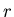be natural numbers such that. We putnumbers in a circle, and we are going to remove numbers. We start counting up form the 1 st number, and the-th,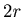-th,-th number, ... are to be removed. We denote bythe position of the last number.

When, then the functionhas very simple recursive relations.

Theorem 1.1andThis is a well known formula. See .
Since, by Theorem1.1 we can calculate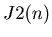for any natural number.

Example 1.1   The graph of the list {J2(n) ,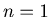, 2, 3, ..., 100}.

Graph 1.1The horizontal coordinate is for the number of numbers involved in the game, and the vertical coordinate is for the last number. For example, bywe have the point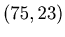in the graph.
As you can see, the graph of the functionis very simple. It is interesting to compare this to the graphs in the following sections.: The Josephus Problem in Both Directions : The Self-Similarity of the Josephus Problem.